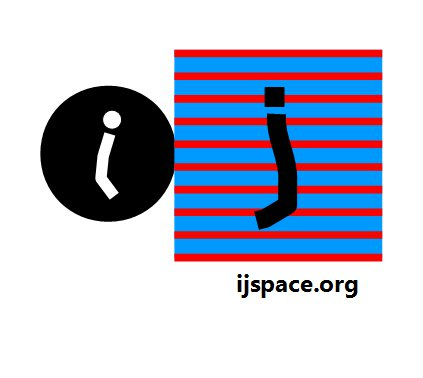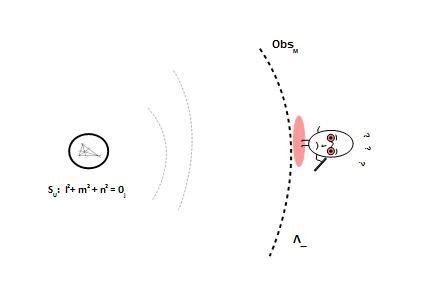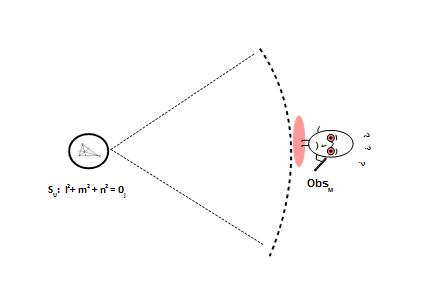# Home

 Stochastic Nature of Quanta-I 25th September 2016 "The idea of a gravitational tensor belongs to the great construction by Einstein. However its definition as given by the Author cannot be considered final. First of all, from the mathematical standpoint, it lacks the invariant character that it should instead necessarily enjoy according to the spirit of general relativity. Even worse is the fact, perceived with keen intuition by Einstein himself, that from such a definition it follows a clearly unacceptable consequence about the gravitational waves. For this point he however finds a way out in quantum theory."..... .....“Since this fact” - these are his words - “should not happen in nature, it seems likely that quantum theory should intervene by modifying not only Maxwell’s electrodynamics, but also the new theory of gravitation”. "Actually there is no need of reaching to quanta......" -T. Levi-Civita Earlier we had discussed some of the ideas behind non-locality and how they relate to EPR-paradox.  But we are yet to discuss the underlying problem which has led to this paradox i.e. whether quantum mechanics provides a complete description of the physical world.  We will try to define what complete description really means in a discrete measurement space or j-space.  We assume that:  The measurements are made by an macroscopic observer ObsM in ʌ∞-plane, The VT-symmetry exists, and The Locality condition (v/c << 1) applies to the observer ObsM. Let us now bring in the concept of Unit-point sphere we have discussed in detail earlier, as shown below:The observer ObsM is situated in Λ∞-plane. If the capacity of the observer ObsM was increased to v/c =1 then observer will be able to measure all the information represented by the Unit-point Sphere SU.     For the capacity v/c<<1, the information represented by the measurements of ObsM is an infinitesimal fraction of the information represented by SU.  The situation is shown in the following diagram:And if we really think about it, this how it actually is:       The infinite information in the measurement metric of ObsM is less than the information contained on the infinitesimal region on the Unit-point sphere.  We need to keep in mind that the following hold true on j-space:   We are in measurement space not in calculation space.  Therefore only the quantities which we can measure have physical significance.  An example is the equation for the Unit-point sphere l2+m2+n2 = 0j1.  The quantity on the right 0j  represents the square of radius or r2.  The calculated quantity √0j or r, has no physical significance.  At best we can consider calculated values such √0j, as an approximation made by the observer in its measurement metric.  The measured physical effects corresponding to relativistic limits, will be of the nature corresponding to fn(r2) or e.g. surface of a sphere 4πr2.  Examples would be nature of charge or gravity.  Since r of SU, can not be measured, the quantity r×r2 has no physical significance2. The position of all the normals in the measurement metric for ObsM will always align with respect to the Unit-point Sphere.  What it means is that the observer ObsM may think that different locations (t,x,y,z) of the measurement space have different directions for the corresponding normals.  But these normals are created with respect to the ObsM's own definition of the origin using VT-symmetry.  For ObsM, all these normals will always be parallel to the normal defined with respect to origin defined in a higher information space. It may seem contradictory in a sense.  However ObsM has very limited capacity, hence it can not measure SU.  If it can not measure SU , then it can not measure the relationship between its own defined origin and SU.  This difference can only be measured by Obsi. Finally the directions of tangents, critically important for the structure formation, are orthogonal to the normals in the measurement metric of the macroscopic observer ObsM.     The most elementary form of the measurement is counting.  Everything else follows afterwards.  For example consider radiation or current.  In either case we count a fundamental unit and then correlate it to the entropy based parameter, "time".  In j-space there are two important aspects of counting based measurements.  (i) The larger the number of measurements for more accurate the estimate is and more importantly  (ii) it is multi-variate (n) problem with n → ∞j.  For ObsM the sample space for the measurement of each variable itself, is infinite.  In such scenario, measurements can not be completely accurate.  Therefore the ObsM can make an estimate at best and that too for a very limited amount of information.  In that case how do we understand the correlation between j-space and the wavefunction ψ? Also how accurate is the statement ∫ψ*ψ dX = 1, in j-space? To be continued...........   ___________________ 1. The Unit-point sphere SU is generated using Pauli's Spin Matrices and therefore represents the measurements made by the observer in relativistic frame, i.e. Obsc. 2.. The volume, fn(r3), related physical effects (e.g. dielectrics), correspond to lower information states. These structures will be measured by the higher capacity observer Obsc (v/c ~ 1) as δ-functions. (Photons would know exactly where to go.)Previous Blogs:   EPR Paradox-I  Nutshell-2015 Chiral Symmetry Sigma-z and I Spin Matrices Rationale behind Irrational Numbers The Ubiquitous z-Axis Majorana ZFC Axioms Set Theory Nutshell-2014 Knots in j-Space Supercolliders Force Riemann Hypothesis Andromeda Nebula Infinite Fulcrum Cauchy and Gaussian Distributions Discrete Space, b-Field & Lower Mass Bound Incompleteness II The Supersymmetry The Cat in Box The Initial State and Symmetries Incompleteness I Discrete Measurement Space The Frog in Well Visual Complex Analysis The Einstein Theory of Relativity

Best viewed with Internet Explorer